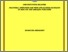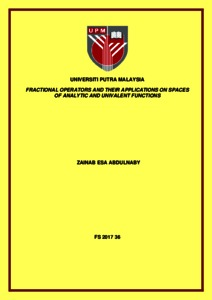# Fractional operators and their applications on spaces of analytic and univalent functions

## Citation

Abdulnaby, Zainab Esa (2017) Fractional operators and their applications on spaces of analytic and univalent functions. Doctoral thesis, Universiti Putra Malaysia.

## Abstract

Fractional calculus operators and linear (or convolution) operators have many interesting applications in the theory of analytic and univalent functions. These operators and their generalizations have been applied in obtaining the characterization properties, coefficient estimates and distortion inequalities for various subclasses of analytic and univalent functions. The main objective of this thesis is to study certain different types of operators such as fractional differential, fractional integral and fractional mixed intgro-differential operators as well as convolution operators besides some classes defined by these operators. Our focus is on spaces of normalized analytic functions in the open unit disk, such as Banach spaces. Firstly, a class of analytic functions with negative coefficients is investigated by constructing the fractional differential operator. Further, we illustrate some of its general geometric properties. We investigate the boundedness and the maximality of the extended fractional differential operator. The mixed integro-differential operator and its generalizations are established. In addition, applications are designed involving theper-Shwarzian derivatives. Moreover, the fractional integral operator is introduced for joining some special functions. Here, we concern about its univalency. Boundednessand compactness on a class of normalized Banach space are discussed. A generalized fractional differential operator and its normalized formula are developed and studied.The above studies are constructed in various types of Banach spaces in a complex domain. Defining the new linear operators associated with the well known special functions such as Mittag-Leffler function are investigated by utilizing the convolution techniques in a complex domain. Studying some bounded transformation operators from Banachto another Banach spaces also, are considered. Extending the Moment-generating function in the complex planeCis applied to define new linear operators of convolution and fractional convolution types which are the generalization for the prominent operators in the open unit disk. Some applications involving these operators are obtained to solve some well known geometric problems such as Fekete–Szeg ̈o problem, while another are solved by using Jack’s Lemma. Finally, we defined a new fractional class of analytic and univalent functions in the open unit disk which can be considered as a generalization of Koebe function. As applications in this class, we defined subclasses of analytic and univalent functions of fractional powers, through employing a fractional differential operator. Then, some certain results on coefficient inequality, growth and distortion theorem and extreme points are studied.Preview
Text
FS 2017 36 IR.pdfView Item